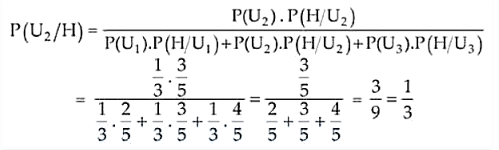Deepak Scored 45->99%ile with Bounce Back Crack Course. You can do it too!

# There are three urns containing 2 white and 3 black balls,

Question:

There are three urns containing 2 white and 3 black balls, 3 white and 2 black balls, and 4 white and 1 black balls, respectively. There is an equal probability of each urn being chosen. A ball is drawn at random from the chosen urn and it is found to be white. Find the probability that the ball drawn was from the second urn.

Solution:

Given, we have 3 urns:

Urn 1 = 2 white and 3 black balls

Urn 2 = 3 white and 2 black balls

Urn 3 = 4 white and 1 black balls

Now, the probabilities of choosing either of the urns are

P(U1) = P(U2) = P(U3) = 1/3

Let H be the event of drawing white ball from the chosen urn.

So,

P(H/U1) = 2/5, P(H/U2) = 3/5 and P(H/U3) = 4/5

By using Baye’s Theorem, we haveTherefore, the required probability is 1/3.